560次阅读## 使用减号函数

``=减去（20,15）``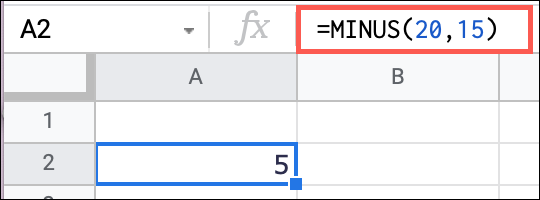``=减去（A2，A3）``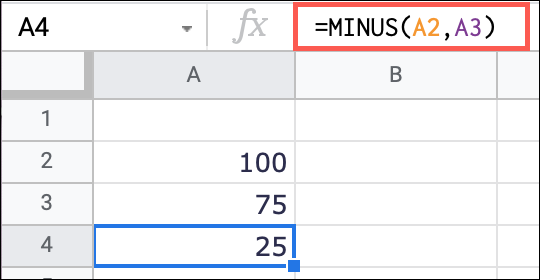## 使用减号

``=20-15``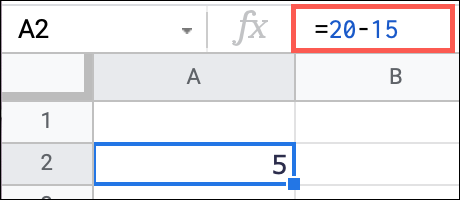``=A2-A3``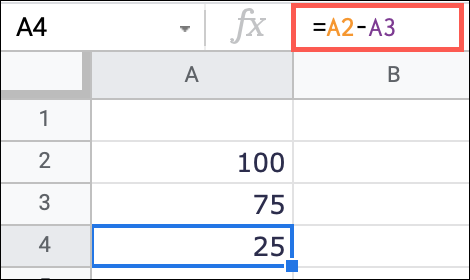## 使用 SUM 函数

``=总和（20-15）``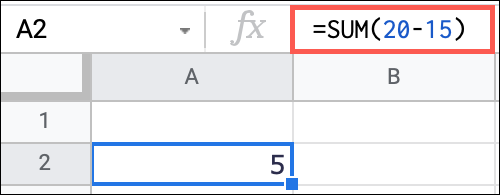``=总和（A2-A3）``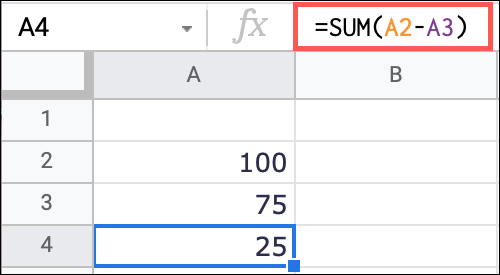## 创建减法方程

``=减去（A2，A3）* 10````=（25-10）+15````=SUM(A2:A5)-A6``